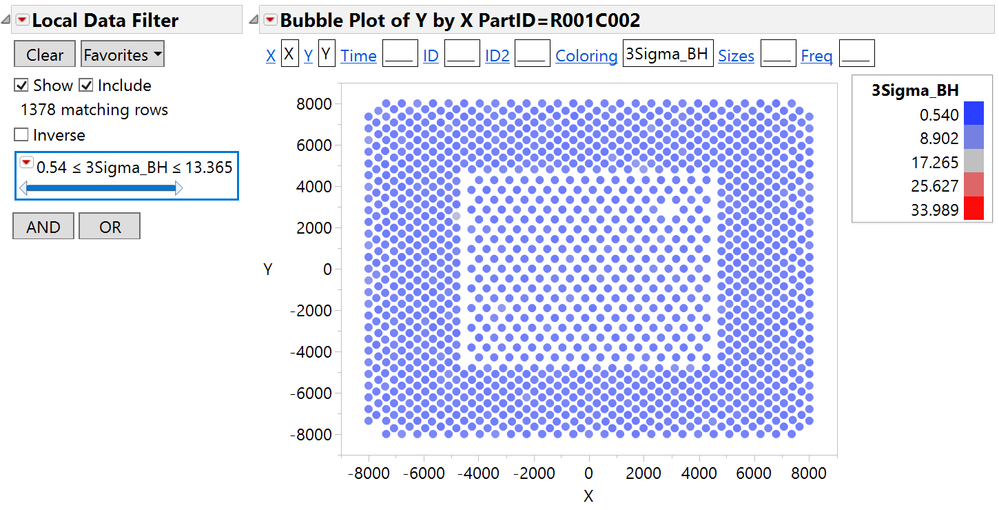Our World Statistics Day conversations have been a great reminder of how much statistics can inform our lives. Do you have an example of how statistics has made a difference in your life? Share your story with the Community!
Choose Language Hide Translation Bar
Highlighted

## Bubble Plot - Set a Dynamic color scale for each column subset

Hello,

I'm writing my very first jmp script and need some help.

So far I started off creating bubble-plots to better visualise my data repeatability distribution in X and Y coordinates for each part I measured.

``````Bubble Plot(
X( :X ),
Y( :Y ),
Coloring( :Name( "3Sigma_BH" ) ),
Show Roles( 1 ),
Color Levels( [0.54 8.902325 17.26465 25.626975 33.9893] ),
Title Position( 0, 0 ),
Local Data Filter(
columns( :Name( "3Sigma_BH" ) ),
Where( :Name( "3Sigma_BH" ) >= 0.54 & :Name( "3Sigma_BH" ) <= 33.9893 )
)
),
By( :PartID ),
SendToReport(
Dispatch(
{},
"1",
ScaleBox,
{Format( "Best", 10 ), Min( -9000 ), Max( 9000 ), Inc( 2000 ),
Minor Ticks( 1 )}
),
Dispatch(
{},
"2",
ScaleBox,
{Format( "Best", 10 ), Min( -9000 ), Max( 9000 ), Inc( 2000 ),
Minor Ticks( 1 )}
)
)
);``````

My problem is with the color scale, I'd like it to dynamically range from each part's min to max.

The variable I try to capture is 3Sigma_BH (which I use for coloring), my column I use for subsetting is PartID. Because of this fixed color-scale I loose context, cfr pic belowI thought of either:

1. Subset my dt by partID before plotting and create a simple function to loop through all partID's at each iteration adding a new bubble plot
2. Create a function to extract the minimum for my current
``````	dtData = currentdatatable()        for each :PartID in dtData (
rows = dtData << get min( :3Sigma_BH)
minBH = Min( :height[rows] );
maxBH = Max( :height[rows] );       ...``````

Any suggestion on how to do this and/or better options than the two above?

Thanks!

Diego

Highlighted

## Re: Bubble Plot - Set a Dynamic color scale for each column subset

Hi Diego - from the red-triangle menu, try turning off "Lock Scales".  This option applies to both the axis scales and the color scales, and can also be set as a preference if you always want this behavior.  With the setting turned off, the Color Levels() and SendToReport() parts of your script should go away when you re-save the script.  Here's an example from the sample data:

``````Open("\$SAMPLE_DATA/San Francisco Crime.jmp");
Bubble Plot(
X( :Longitude ),
Y( :Latitude ),
Coloring( :Time ),
Lock Scales( 0 ),
Title Position( 0, 0 ),
Local Data Filter(